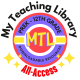Math

# Math Glossary – A Great Student Resource!

This 38 page math glossary has been designed for 3rd-5th grades and includes 129 math terms with definitions and 72 illustrations! Terms include those surrounding basic operations, geometry and elementary statistics.

Covers:

1. < and >
2. 12-hour and 24-hour clock
3. 2D shapes
4. 3D shapes
5. acute angle
6. analog and digital clocks
7. area
8. array
9. ascending order
10. associative property
11. average
12. axis
13. bar chart
14. bar model
15. block graph
16. BODMAS
17. bridging through 10
18. bus stop method
19. capacity
20. cardinal numbers
21. Carroll diagram
22. chunking
23. circle
24. circumference
25. clockwise and counterclockwise
26. coordinates
27. column method
28. commutative property
30. converting into same units
31. cube numbers
32. data handling
33. decimal
34. degrees
35. denominator
36. descending order
37. diagonal
38. diameter
39. distributive property
40. division facts
41. edge
42. equation
43. equilateral triangle
44. equivalent fractions and simplifying fractions
45. estimate
46. expanded notation
47. face
48. factor
49. finding the difference between two numbers
50. formula
51. greatest common factor
52. grid method horizontal improper fraction
53. integer
54. inverse operation
55. isosceles triangle
56. least common denominator
57. least common multiple
58. line graph
59. long division
60. long multiplication
61. mass
62. means
63. median
64. mirror line
65. mixed number
66. mode
67. multiple
68. negative numbers
69. net
70. number bonds
71. number facts
72. number line and number ladder
73. number sentence
74. number square
75. numerator
76. obtuse angle
77. odd and even numbers
78. operation
79. ordinal numbers
80. parallel
81. partitioning
82. percentage
83. perimeter
84. perpendicular
85. pictogram
86. pie chart
87. place value
88. polygon
89. prime number
90. prism
91. probability
92. product
93. proportion
94. pyramid
97. range
98. ratio
99. reflection of shapes
100. reflective symmetry
101. reflex angle
102. regular and irregular shapes
103. right angle
104. right-angled triangle
105. roman numeral
106. rotation of shapes
107. rotational symmetry
108. rounding numbers
110. scale factor
111. scalene triangle
112. square numbers
113. standard and non-standard units
114. sum
115. symmetry
116. tally chart
117. tessellation
118. time intervals
119. translation of shapes
120. triangle
121. triangular numbers
122. turns
123. two-step and multi-step problems
124. unit fractions
125. Venn diagram
126. vertex / vertices
127. vertical
128. volume
129. word problem or story problem

BE the FIRST to know about NEW Products by joining the…

My Teaching Library FB group
Posts are made in this group first!!

Subscribe to My Teaching Library newsletters!

## 4 thoughts on “Math Glossary – A Great Student Resource!”

1.L says:

Great resource, thanks for sharing!

1.My Teaching Library / CHSH-Teach, LLC says:

You are welcome!

2.Caylissa says:

This is a great math resource!

1.My Teaching Library / CHSH-Teach, LLC says:

Thank you Caylissa!